# Selina Solutions Concise Mathematics Class 6 Chapter 8: H.C.F and L.C.M Exercise 8(A)

Selina Solutions Concise Mathematics Class 6 Chapter 8 H.C.F and L.C.M Exercise 8(A) helps students in solving the problems easily, which are important from an exam point of view. Students who find obstacles in grasping the topics covered are advised to use Selina Solutions to understand in-depth. Expert teachers have designed these solutions in such a way that students who practice frequently succeed in clearing their doubts immediately. For better conceptual knowledge, students can download Selina Solutions Concise Mathematics Class 6 Chapter 8 H.C.F and L.C.M Exercise 8(A) PDF, from the links provided below

## Selina Solutions Concise Mathematics Class 6 Chapter 8: H.C.F and L.C.M Exercise 8(A) Download PDF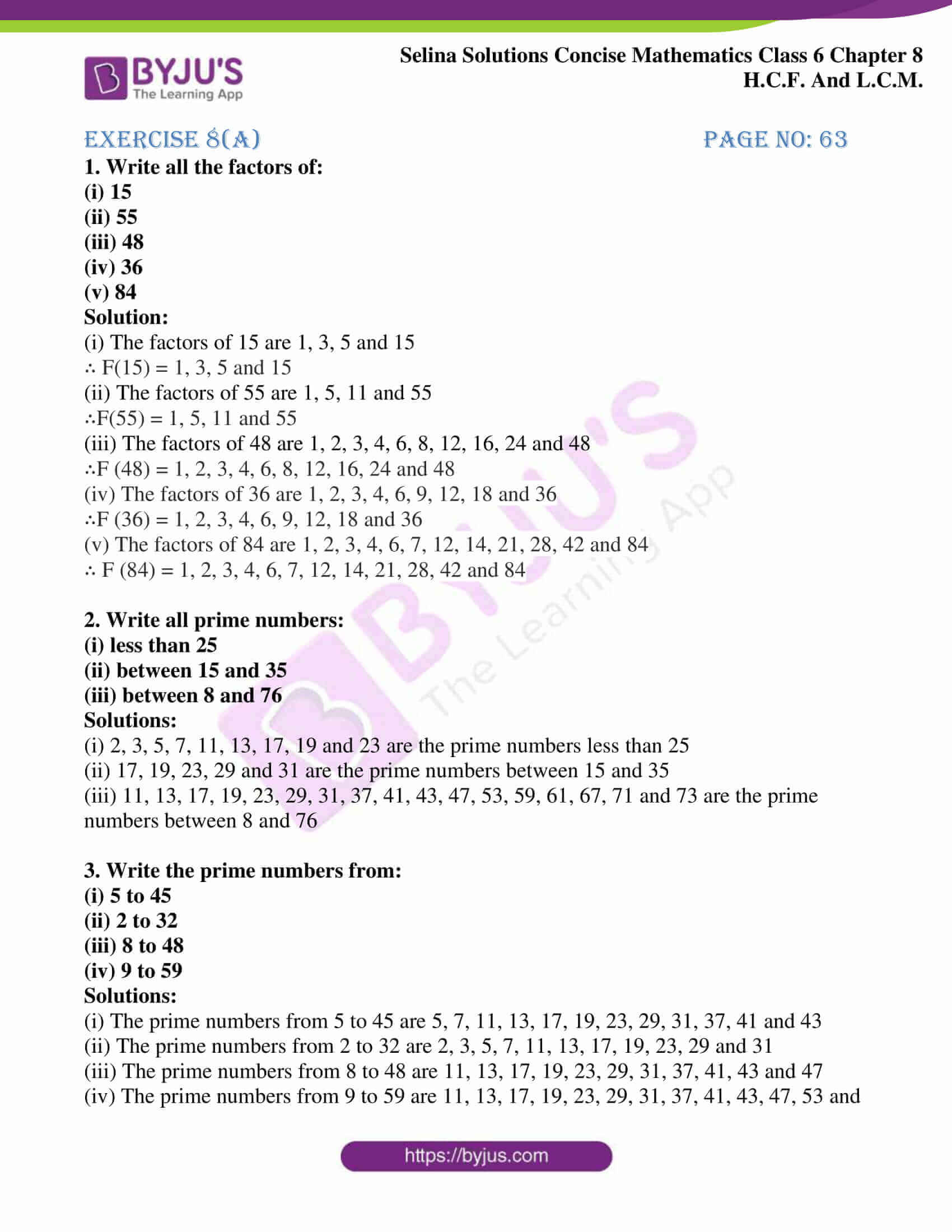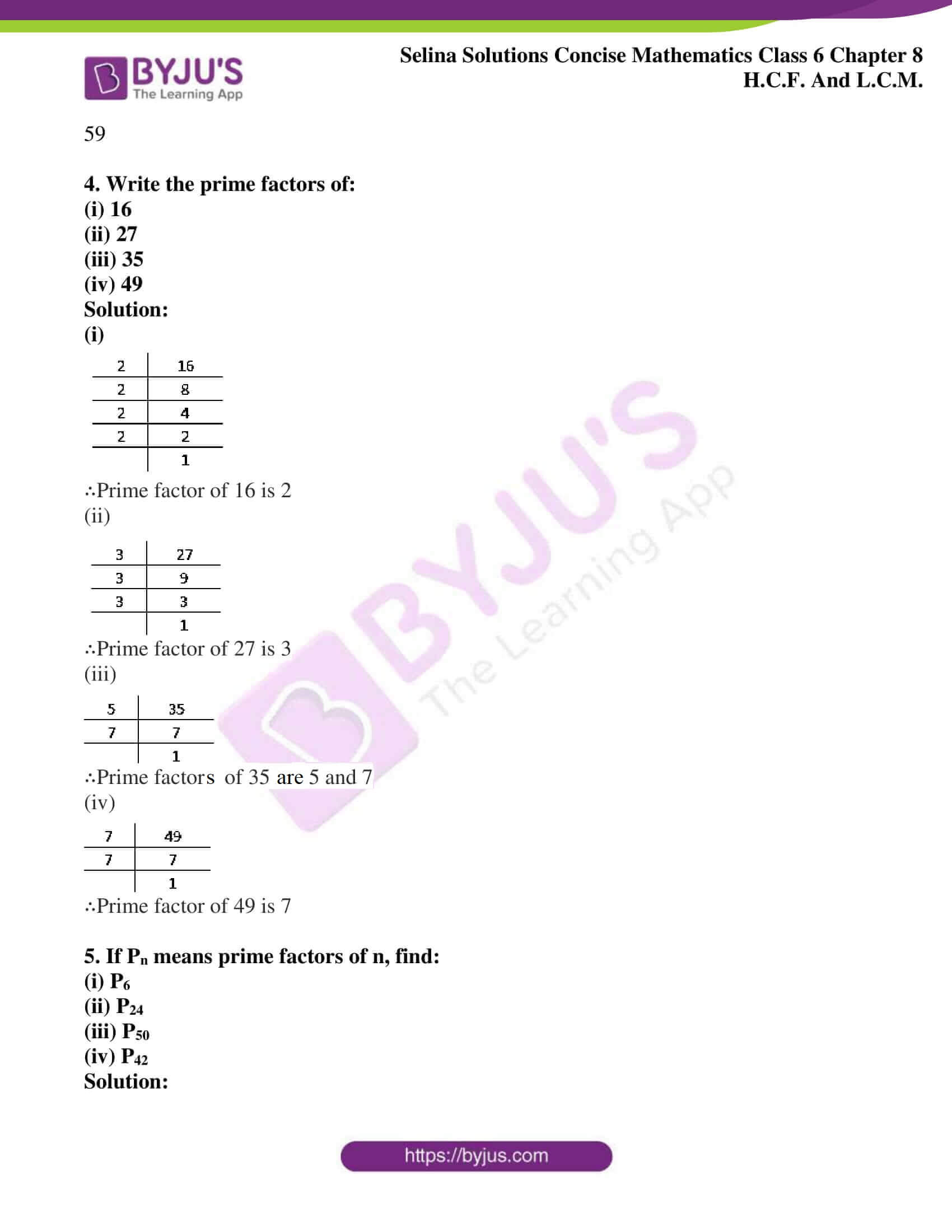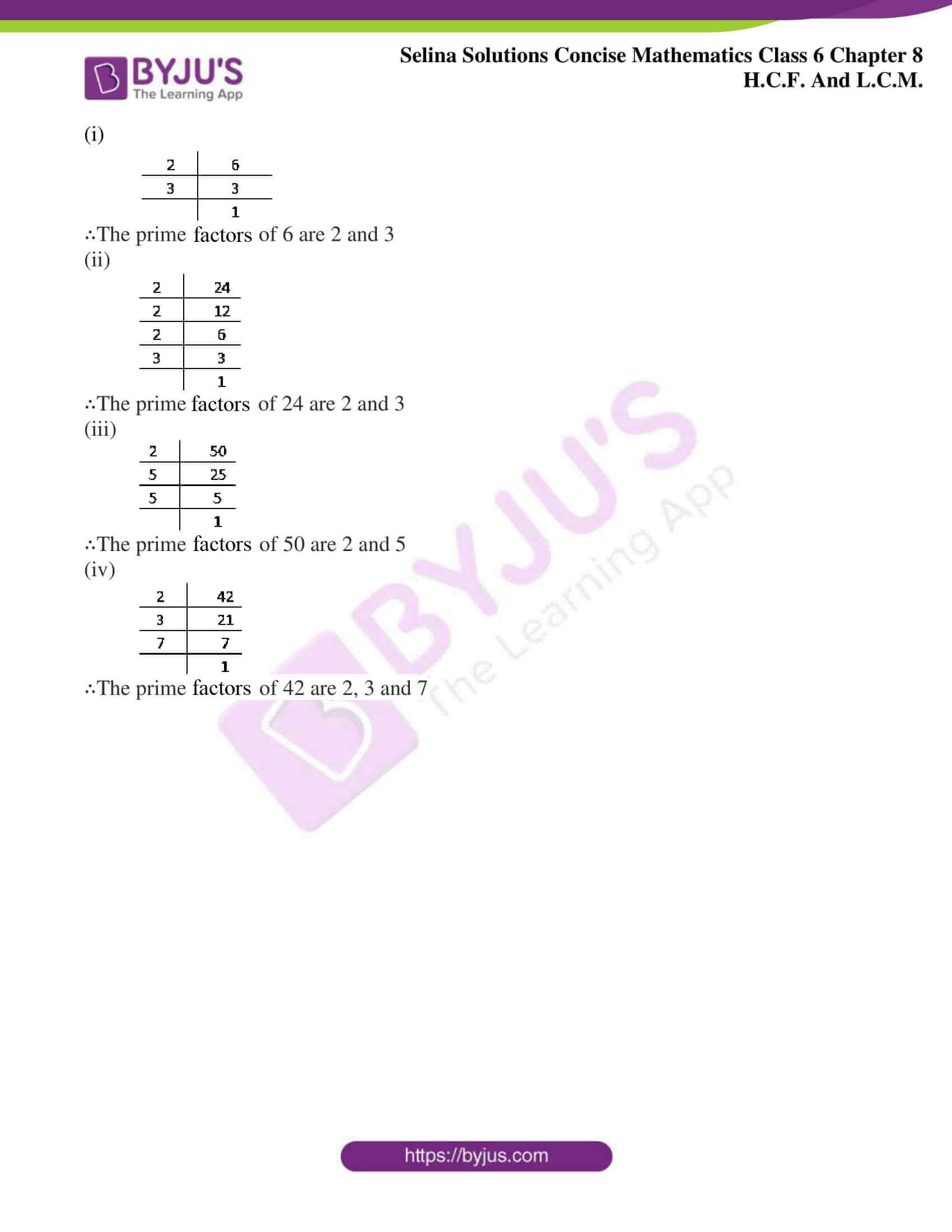### Access other exercises of Selina Solutions Concise Mathematics Class 6 Chapter 8: H.C.F and L.C.M

Exercise 8(B) Solutions

Exercise 8(C) Solutions

### Access Selina Solutions Concise Mathematics Class 6 Chapter 8: H.C.F and L.C.M Exercise 8(A)

#### Exercise 8(A) page No: 63

1. Write all the factors of:

(i) 15

(ii) 55
(iii) 48

(iv) 36

(v) 84

Solution:

(i) The factors of 15 are 1, 3, 5 and 15

∴ F(15) = 1, 3, 5 and 15

(ii) The factors of 55 are 1, 5, 11 and 55

∴F(55) = 1, 5, 11 and 55

(iii) The factors of 48 are 1, 2, 3, 4, 6, 8, 12, 16, 24 and 48

∴F (48) = 1, 2, 3, 4, 6, 8, 12, 16, 24 and 48

(iv) The factors of 36 are 1, 2, 3, 4, 6, 9, 12, 18 and 36

∴F (36) = 1, 2, 3, 4, 6, 9, 12, 18 and 36

(v) The factors of 84 are 1, 2, 3, 4, 6, 7, 12, 14, 21, 28, 42 and 84

∴ F (84) = 1, 2, 3, 4, 6, 7, 12, 14, 21, 28, 42 and 84

2. Write all prime numbers:

(i) less than 25

(ii) between 15 and 35

(iii) between 8 and 76

Solutions:

(i) 2, 3, 5, 7, 11, 13, 17, 19 and 23 are the prime numbers less than 25

(ii) 17, 19, 23, 29 and 31 are the prime numbers between 15 and 35

(iii) 11, 13, 17, 19, 23, 29, 31, 37, 41, 43, 47, 53, 59, 61, 67, 71 and 73 are the prime numbers between 8 and 76

3. Write the prime numbers from:

(i) 5 to 45

(ii) 2 to 32

(iii) 8 to 48

(iv) 9 to 59

Solutions:

(i) The prime numbers from 5 to 45 are 5, 7, 11, 13, 17, 19, 23, 29, 31, 37, 41 and 43

(ii) The prime numbers from 2 to 32 are 2, 3, 5, 7, 11, 13, 17, 19, 23, 29 and 31

(iii) The prime numbers from 8 to 48 are 11, 13, 17, 19, 23, 29, 31, 37, 41, 43 and 47

(iv) The prime numbers from 9 to 59 are 11, 13, 17, 19, 23, 29, 31, 37, 41, 43, 47, 53 and 59

4. Write the prime factors of:

(i) 16

(ii) 27

(iii) 35

(iv) 49

Solution:

(i)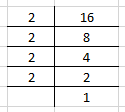∴Prime factor of 16 is 2

(ii)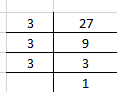∴Prime factor of 27 is 3

(iii)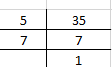∴Prime factors of 35 are 5 and 7

(iv)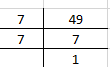∴Prime factor of 49 is 7

5. If Pn means prime factors of n, find:

(i) P6

(ii) P24

(iii) P50

(iv) P42

Solution:

(i)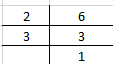∴The prime factors of 6 are 2 and 3

(ii)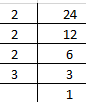∴The prime factors of 24 are 2 and 3

(iii)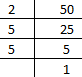∴The prime factors of 50 are 2 and 5

(iv)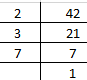∴The prime factors of 42 are 2, 3 and 7